# Efficient In-Memory Data Analytics with Python and pandas¶

Dr. Yves J. Hilpisch

The Python Quants GmbH

www.pythonquants.com

yves@pythonquants.com

@dyjh

CodeJam, Forschungszentrum Jülich – 29. Januar 2014

A brief bio:

• Managing Partner of The Python Quants
• Founder of Visixion GmbH – The Python Quants
• Lecturer Mathematical Finance at Saarland University
• Focus on Financial Industry and Financial Analytics
• Book (2013) "Derivatives Analytics with Python"
• Book (July 2014) "Python for Finance", O'Reilly
• Dr.rer.pol in Mathematical Finance
• Martial Arts Practitioner and Fan

## Python for Analytics¶

Corporations, decision makers and analysts nowadays generally face a number of problems with data:

• sources: data typically comes from different sources, like from the Web, from in-house databases or it is generated in-memory, e.g. for simulation purposes
• formats: data is generally available in different formats, like SQL databases/tables, Excel files, CSV files, arrays, proprietary formats
• structure: data typically comes differently structured, be it unstructured, simply indexed, hierarchically indexed, in table form, in matrix form, in multidimensional arrays
• completeness: real-world data is generally incomplete, i.e. there is missing data (e.g. along an index) or multiple series of data cannot be aligned correctly (e.g. two time series with different time indexes)
• conventions: for some types of data there a many “competing” conventions with regard to formatting, like for dates and time
• interpretation: some data sets contain information that can be easily and intelligently interpreted, like a time index, others not, like texts
• performance: reading, streamlining, aligning, analyzing – i.e. processing – (big) data sets might be slow

In addition to these data-oriented problems, there typically are organizational issues that have to be considered:

• departments: the majority of companies is organized in departments with different technologies, databases, etc., leading to “data silos”
• analytics skills: analytical and business skills in general are possessed by people working in line functions (e.g. production) or administrative functions (e.g. finance)
• technical skills: technical skills, like retrieving data from databases and visualizing them, are generally possessed by people in technology functions (e.g. development, systems operations)

This presentation focuses on

• Python & pandas as a general purpose data analytics environment
• interactive analytics examples
• prototyping-like Python usage

It does not address such important issues like

• architectural issues regarding hardware and software
• development processes, testing, documentation and production
• real world problem modeling

## Some Python Fundamentals¶

### Fundamental Python Libraries¶

A fundamental Python stack for interactive data analytics and visualization should at least contain the following libraries tools:

• Python – the Python interpreter itself
• NumPy – high performance, flexible array structures and operations
• SciPy – collection of scientific modules and functions (e.g. for regression, optimization, integration)
• pandas – time series and panel data analysis and I/O
• PyTables – hierarchical, high performance database (e.g. for out-of-memory analytics)
• matplotlib – 2d and 3d visualization
• IPython – interactive data analytics, visualization, publishing

It is best to use either the Python distribution Anaconda or the Web-based analytics environment Wakari. Both provide almost "complete" Python environments.

For example, pandas can, among others, help with the following data-related problems:

• sources: pandas reads data directly from different data sources such as SQL databases, Excel files or JSON based APIs
• formats: pandas can process input data in different formats like CSV files or Excel files; it can also generate output in different formats like CSV, XLS, HTML or JSON
• structure: pandas' strength lies in structured data formats, like time series and panel data
• completeness: pandas automatically deals with missing data in most circumstances, e.g. computing sums even if there are a few or many “not a number”, i.e. missing, values
• conventions/interpretation: for example, pandas can interpret and convert different date-time formats to Python datetime objects and/or timestamps
• performance: the majority of pandas classes, methods and functions is implemented in a performance-oriented fashion making heavy use of the Python/C compiler Cython

### First Analytics Steps with Python¶

As a simple example let's generate a NumPy array with five sets of 1000 (pseudo-)random numbers each.

In :
import numpy as np  # this imports the NumPy library

In :
data = np.random.standard_normal((5, 1000))  # generate 5 sets with 1000 rn each
data[:, :5].round(3)  # print first five values of each set rounded to 3 digits

Out:
array([[ 0.785, -0.544,  0.437,  0.793, -1.705],
[-0.476, -0.115, -0.95 , -0.8  ,  0.172],
[-0.349, -1.35 ,  0.188, -0.521, -1.299],
[ 0.392,  0.062,  0.755, -1.712,  0.966],
[-0.252, -0.504,  1.731, -0.559,  1.605]])


Let's plot a histogram of the 1st, 2nd and 3rd data set.

In :
import matplotlib.pyplot as plt  # this imports matplotlib.pyplot

In :
plt.hist([data, data, data], label=['Set 0', 'Set 1', 'Set 2'])
plt.grid(True)  # grid for better readability
plt.legend()

Out:
<matplotlib.legend.Legend at 0x105cc5910>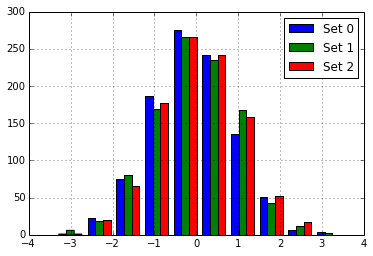We then want to plot the 'running' cumulative sum of each set.

In :
plt.figure()  # initialize figure object
plt.grid(True)
for data_set in enumerate(data):  # iterate over all rows
plt.plot(data_set.cumsum(), label='Set %s' % data_set)
# plot the running cumulative sums for each row
plt.legend(loc=0)  # write legend with labels

Out:
<matplotlib.legend.Legend at 0x105f2ffd0>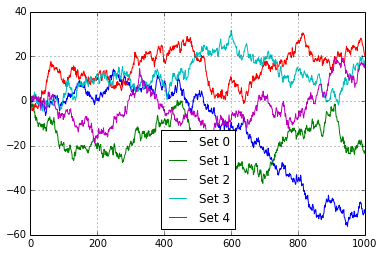Some fundamental statistics from our data sets.

In :
data.mean(axis=1)  # average value of the 5 sets

Out:
array([-0.05009487, -0.02224559,  0.01991948,  0.01816627,  0.01484693])

In :
data.std(axis=1)  # standard deviation of the 5 sets

Out:
array([ 0.97038583,  1.00002371,  0.96460241,  1.01051799,  0.99002334])

In :
np.round(np.corrcoef(data), 2)  # correlation matrix of the 5 data sets

Out:
array([[ 1.  , -0.01,  0.03,  0.02, -0.05],
[-0.01,  1.  , -0.02, -0.04,  0.08],
[ 0.03, -0.02,  1.  ,  0.04, -0.03],
[ 0.02, -0.04,  0.04,  1.  ,  0.04],
[-0.05,  0.08, -0.03,  0.04,  1.  ]])


## Data Analytics with Python & pandas¶

### Creating a Database to Work With¶

Currently, and in the foreseeable future as well, most corporate data is stored in SQL databases. We take this as a starting point and generate a SQL table with dummy data.

In :
filename = 'numbers'
import sqlite3 as sq3  # imports the SQLite library
# Remove files if they exist
import os
try:
os.remove(filename + '.db')
os.remove(filename + '.h5')
os.remove(filename + '.csv')
os.remove(filename + '.xlsx')
except:
pass


We create a table and populate the table with the dummy data.

In :
query = 'CREATE TABLE numbs (no1 real, no2 real, no3 real, no4 real, no5 real)'
con = sq3.connect(filename + '.db')
con.execute(query)
con.commit()

In :
# Writing Random Numbers to SQLite3 Database
rows = 500000  # 500,000 rows for the SQL table (ca. 25 MB in size)
nr = np.random.standard_normal((rows, 5))
%time con.executemany('INSERT INTO numbs VALUES(?, ?, ?, ?, ?)', nr)
con.commit()

CPU times: user 8.41 s, sys: 176 ms, total: 8.59 s
Wall time: 8.62 s



### Reading Data into NumPy Arrays¶

We can now read the data into a NumPy array.

In :
# Reading a couple of rows from SQLite3 database table
array(con.execute('SELECT * FROM numbs').fetchmany(3)).round(3)

Out:
array([[ 2.322, -1.008,  0.967,  0.84 ,  0.565],
[-0.708, -0.826, -0.042, -1.043,  2.123],
[-1.141, -0.388, -1.418,  1.172,  0.093]])

In :
# Reading the whole table into an NumPy array
%time result = array(con.execute('SELECT * FROM numbs').fetchall())

CPU times: user 4.85 s, sys: 155 ms, total: 5 s
Wall time: 5.06 s


In :
result[:3].round(3)  # the same rows from the in-memory array

Out:
array([[ 2.322, -1.008,  0.967,  0.84 ,  0.565],
[-0.708, -0.826, -0.042, -1.043,  2.123],
[-1.141, -0.388, -1.418,  1.172,  0.093]])

In :
nr = 0.0; result = 0.0  # memory clean-up


pandas is optimized both for convenience and performance when it comes to analytics and I/O operations.

In :
import pandas as pd
import pandas.io.sql as pds

In :
%time data = pds.read_frame('SELECT * FROM numbs', con)
con.close()

CPU times: user 1.93 s, sys: 132 ms, total: 2.06 s
Wall time: 2.07 s


In :
data.head()

Out:
no1 no2 no3 no4 no5
0 2.321564 -1.007644 0.966935 0.839818 0.565468
1 -0.707621 -0.825653 -0.042492 -1.042881 2.122805
2 -1.141395 -0.388145 -1.418309 1.172099 0.093294
3 -1.656099 0.111377 -1.839475 -0.365148 0.162408
4 0.112878 -1.655273 -0.508052 -0.287389 -0.559542

5 rows × 5 columns

The plotting of larger data sets often is a problem. This can easily be accomplished here by only plotting every 500th data row, for instance.

In :
data[::500].cumsum().plot(grid=True, legend=True)  # every 500th row is plotted

Out:
<matplotlib.axes.AxesSubplot at 0x108758450>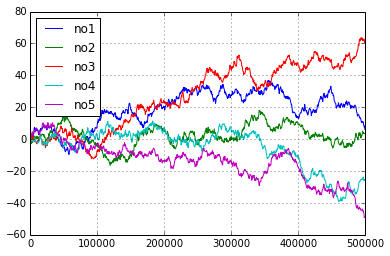### Writing Data with pandas¶

There are some reasons why you may want to write the DataFrame to disk:

• Database Performance: you do not want to query the SQL database everytime anew
• IO Speed: you want to increase data/information retrievel
• Data Structure: maybe you want to store additional data with the original data set or you have generated a DataFrame out of multiple sources

pandas is tightly integrated with PyTables, a library that implements the HDF5 standard for Python. It makes data storage and I/O operations not only convenient but quite fast as well.

In :
h5 = pd.HDFStore(filename + '.h5', 'w')
%time h5['data'] = data  # writes the DataFrame to HDF5 database

CPU times: user 28.9 ms, sys: 35.9 ms, total: 64.8 ms
Wall time: 75.6 ms



Now, we can read the data from the HDF5 file into another DataFrame object. Performance of such an operation generally is quite high.

In :
%time data_new = h5['data']

CPU times: user 3.29 ms, sys: 12.6 ms, total: 15.9 ms
Wall time: 14.9 ms


In :
%time data.sum() - data_new.sum()   # checking if the DataFrames are the same

CPU times: user 73.6 ms, sys: 13.2 ms, total: 86.8 ms
Wall time: 83.6 ms


Out:
no1    0
no2    0
no3    0
no4    0
no5    0
dtype: float64

In :
%time np.allclose(data, data_new, rtol=0.1)

CPU times: user 85.5 ms, sys: 28.6 ms, total: 114 ms
Wall time: 113 ms


Out:
True

In :
data_new = 0.0  # memory clean-up
h5.close()


### In-Memory Analytics with pandas¶

pandas has powerful selection and querying mechanisms (I).

In :
res = data[['no1', 'no2']][((data['no2'] > 0.5) | (data['no2'] < -0.5))]

In :
plt.plot(res.no1, res.no2, 'ro')
plt.grid(True); plt.axis('tight')

Out:
(-4.5855019802135892,
4.2902614658186531,
-4.7115453577460711,
4.5006018407498072)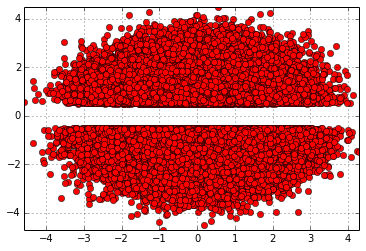pandas has powerful selection and querying mechanisms (II).

In :
res = data[['no1', 'no2']][((data['no1'] > 0.5) | (data['no1'] < -0.5))
& ((data['no2'] < -1) | (data['no2'] > 1))]

In :
plt.plot(res.no1, res.no2, 'ro')
plt.grid(True); plt.axis('tight')

Out:
(-4.360512668462273,
4.2902614658186531,
-4.7115453577460711,
4.5006018407498072)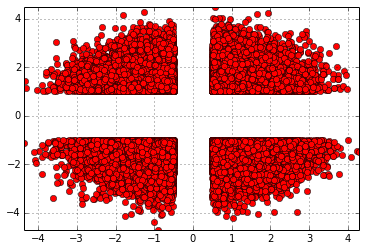pandas has powerful selection and querying mechanisms (III).

In :
res = data[['no1', 'no2', 'no3' ]][((data['no1'] > 1.5) | (data['no1'] < -1.5))
& ((data['no2'] < -1) | (data['no2'] > 1))
& ((data['no3'] < -1) | (data['no3'] > 1))]

In :
from mpl_toolkits.mplot3d import Axes3D
fig = plt.figure(figsize=(8, 5))
ax.scatter(res.no1, res.no2, res.no3, zdir='z', s=25, c='r', marker='o')
ax.set_xlabel('no1'); ax.set_ylabel('no2'); ax.set_zlabel('no3')

Out:
<matplotlib.text.Text at 0x10616fa10>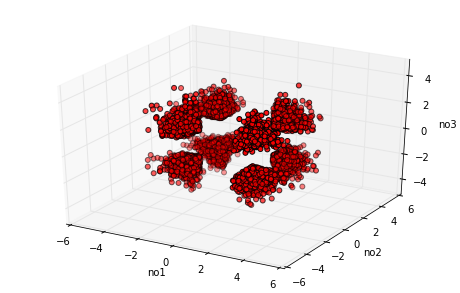### Exporting Data with pandas¶

The data is easily exported to different standard data file formats like comma separated value files (CSV).

In :
%time data.to_csv(filename + '.csv')  # writes the whole data set as CSV file

CPU times: user 5.04 s, sys: 176 ms, total: 5.21 s
Wall time: 5.25 s


In :
csv_file = open(filename + '.csv')
for i in range(5):

,no1,no2,no3,no4,no5
0,2.321564183657276,-1.0076441547182922,0.9669345116776533,0.8398179850987361,0.5654678971576657
1,-0.7076206524338957,-0.8256527633342371,-0.042492017121949534,-1.042880737514809,2.12280463091422
2,-1.1413946947894245,-0.3881445591466267,-1.4183091603968363,1.1720993048812203,0.09329359013480651
3,-1.6560985353714768,0.1113772366765862,-1.8394747939237726,-0.36514754370691044,0.16240810586181725



pandas also interacts easily with Microsoft Excel spreadsheet files (XLS/XLSX).

In :
%time data.ix[:10000].to_excel(filename + '.xlsx')  # first 10,000 rows written in a Excel file

CPU times: user 14.3 s, sys: 295 ms, total: 14.6 s
Wall time: 14.5 s



With pandas you can in one step read data from an Excel file and e.g. plot it.

In :
%time pd.read_excel(filename + '.xlsx', 'Sheet1').hist(grid=True)

CPU times: user 1.17 s, sys: 14.5 ms, total: 1.18 s
Wall time: 1.19 s


Out:
array([[<matplotlib.axes.AxesSubplot object at 0x10e42e190>,
<matplotlib.axes.AxesSubplot object at 0x10dae7fd0>,
<matplotlib.axes.AxesSubplot object at 0x10c17cf50>],
[<matplotlib.axes.AxesSubplot object at 0x10f801b50>,
<matplotlib.axes.AxesSubplot object at 0x10f7fae90>,
<matplotlib.axes.AxesSubplot object at 0x110d6bdd0>]], dtype=object)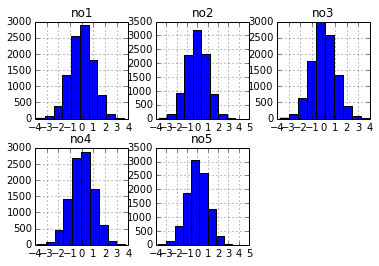One can also generate HTML code, e.g. for automatic Web-based report generation.

In :
html_code = data.ix[:2].to_html()
html_code

Out:
u'<table border="1" class="dataframe">\n  <thead>\n    <tr style="text-align: right;">\n      <th></th>\n      <th>no1</th>\n      <th>no2</th>\n      <th>no3</th>\n      <th>no4</th>\n      <th>no5</th>\n    </tr>\n  </thead>\n  <tbody>\n    <tr>\n      <th>0</th>\n      <td> 2.321564</td>\n      <td>-1.007644</td>\n      <td> 0.966935</td>\n      <td> 0.839818</td>\n      <td> 0.565468</td>\n    </tr>\n    <tr>\n      <th>1</th>\n      <td>-0.707621</td>\n      <td>-0.825653</td>\n      <td>-0.042492</td>\n      <td>-1.042881</td>\n      <td> 2.122805</td>\n    </tr>\n    <tr>\n      <th>2</th>\n      <td>-1.141395</td>\n      <td>-0.388145</td>\n      <td>-1.418309</td>\n      <td> 1.172099</td>\n      <td> 0.093294</td>\n    </tr>\n  </tbody>\n</table>'

In :
from IPython.core.display import HTML

In :
HTML(html_code)

Out:
no1 no2 no3 no4 no5
0 2.321564 -1.007644 0.966935 0.839818 0.565468
1 -0.707621 -0.825653 -0.042492 -1.042881 2.122805
2 -1.141395 -0.388145 -1.418309 1.172099 0.093294

Some final checks and data cleaning.

In :
ll numb*

-rw-rw-rw-  1 yhilpisch  staff  52468263 29 Jan 12:15 numbers.csv
-rw-r--r--  1 yhilpisch  staff  27224064 29 Jan 12:15 numbers.db
-rw-rw-rw-  1 yhilpisch  staff  24007368 29 Jan 12:15 numbers.h5
-rw-rw-rw-  1 yhilpisch  staff    766583 29 Jan 12:15 numbers.xlsx


In :
data = 0.0  # memory clean-up


## N-Body Simulation¶

The simulation of the movement of N bodies in relation to each other, where $N$ is a large number and a body can be a star/planet or a small particle, is an important problem in Physics.

The basic simulation algorithm involves a number of iterations ("loops") since one has to determine the force that every single body exerts on all the other bodies in the system to be simulated (e.g. a galaxy or a system of atomic particles).

The algorithm lends iteself pretty well for parallization. However, the example code that follows does not use any parallelization techniques. It illustrates rather what speed-ups are achievable by

• using vectorization approaches with NumPy and
• just-in-time compiling capabilities of Numba

A description and discussion of the algorithm is found in the recent article Vladimirov, Andrey and Vadim Karpusenko (2013): "Test-Driving Intel Xeon-Phi Coprocessors with a Basic N-Body Simulation." White Paper, Colfax International.

### Pure Python¶

We first use pure Python to implement the algorithm. We start by generating random vectors of particle locations and a zero vector for the speed of each particle.

In :
import random

In :
nParticles = 250
nSteps = 15

In :
p_py = []
for i in range(nParticles):
p_py.append([random.gauss(0.0, 1.0) for j in range(3)])

In :
pv_py = [[0, 0, 0] for x in p_py]


Next, we define the simulation function for the movements of each particle given their gravitational interaction.

In :
def nbody_py(particle, particlev):  # lists as input
dt = 0.01
for step in range(1, nSteps + 1, 1):
for i in range(nParticles):
Fx = 0.0; Fy = 0.0; Fz = 0.0
for j in range(nParticles):
if j != i:
dx = particle[j] - particle[i]
dy = particle[j] - particle[i]
dz = particle[j] - particle[i]
drSquared = dx * dx + dy * dy + dz * dz
drPowerN32 = 1.0 / (drSquared + sqrt(drSquared))
Fx += dx * drPowerN32
Fy += dy * drPowerN32
Fz += dz * drPowerN32
particlev[i] += dt * Fx
particlev[i] += dt * Fy
particlev[i] += dt * Fz
for i in range(nParticles):
particle[i] += particlev[i] * dt
particle[i] += particlev[i] * dt
particle[i] += particlev[i] * dt
return particle, particlev


We then execute the function to measure execution speed.

In :
%time p_py, pv_py = nbody_py(p_py, pv_py)

CPU times: user 13.1 s, sys: 33.9 ms, total: 13.1 s
Wall time: 13.1 s



### NumPy Solution¶

The basic algorithm allows for a number of vectorizations by using NumPy.

In :
import numpy as np

In :
p_np = np.random.standard_normal((nParticles, 3))
pv_np = np.zeros_like(p_np)


Using NumPy arrays and matplotlib's 3d plotting capabilities, we can easily visualize the intial positions of the particles in the space.

In :
fig = plt.figure()
ax.scatter(p_np[:, 0], p_np[:, 1], p_np[:, 2],
c='r', marker='.')

Out:
<mpl_toolkits.mplot3d.art3d.Patch3DCollection at 0x10b360510>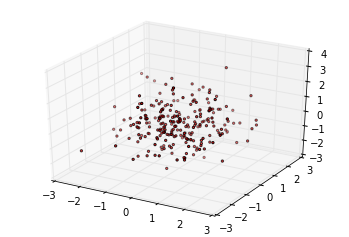The respective NumPy simulation function is considerably more compact.

In :
def nbody_np(particle, particlev):  # NumPy arrays as input
dt = 0.01
for step in range(1, nSteps + 1, 1):
Fp = np.zeros((nParticles, 3))
for i in range(nParticles):
dp = particle - particle[i]
drSquared = np.sum(dp ** 2, axis=1)
h = drSquared + np.sqrt(drSquared)
drPowerN32 = 1. / np.maximum(h, 1E-10)
Fp += -(dp.T * drPowerN32).T
particlev += dt * Fp
particle += particlev * dt
return particle, particlev


It is also considerably faster.

In :
%time p_np, pv_np = nbody_np(p_np, pv_np)

CPU times: user 204 ms, sys: 1.19 ms, total: 205 ms
Wall time: 205 ms



Let's check how the particles moved. The following is a snapshot of the final positions of all particles.

In :
fig = plt.figure()
ax.scatter(p_np[:, 0], p_np[:, 1], p_np[:, 2],
c='r', marker='.')

Out:
<mpl_toolkits.mplot3d.art3d.Patch3DCollection at 0x10b68e1d0>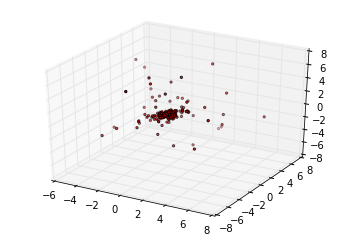### Dynamically Compiled Version¶

For this case, we first implement a function which is somehow a hybrid function of the pure Python (loop-heavy) and the NumPy array class-based version.

In :
def nbody_hy(particle, particlev):  # NumPy arrays as input
dt = 0.01
for step in range(1, nSteps + 1, 1):
for i in range(nParticles):
Fx = 0.0; Fy = 0.0; Fz = 0.0
for j in range(nParticles):
if j != i:
dx = particle[j,0] - particle[i,0]
dy = particle[j,1] - particle[i,1]
dz = particle[j,2] - particle[i,2]
drSquared = dx * dx + dy * dy + dz * dz
drPowerN32 = 1.0 / (drSquared + sqrt(drSquared))
Fx += dx * drPowerN32
Fy += dy * drPowerN32
Fz += dz * drPowerN32
particlev[i, 0] += dt * Fx
particlev[i, 1] += dt * Fy
particlev[i, 2] += dt * Fz
for i in range(nParticles):
particle[i,0] += particlev[i,0] * dt
particle[i,1] += particlev[i,1] * dt
particle[i,2] += particlev[i,2] * dt
return particle, particlev


This function is then compiled with Numba.

In :
import numba as nb

In :
nbody_nb = nb.autojit(nbody_hy)


This new, compiled version of the hybrid function is yet faster.

In :
p_nb = np.random.standard_normal((nParticles, 3))
pv_nb = np.zeros_like(p_nb)

In :
%time p_nb, pv_nb = nbody_nb(p_nb, pv_nb)

CPU times: user 20.5 ms, sys: 33 µs, total: 20.5 ms
Wall time: 20.5 ms



### Speed Comparisons¶

A helper function to compare the performances.

In :
def perf_comp_data(func_list, data_list, rep=3, number=1):
from timeit import repeat
res_list = {}
for name in enumerate(func_list):
stmt = name + '(' + data_list[name] + ')'
setup = "from __main__ import " + name + ', ' + data_list[name]
results = repeat(stmt=stmt, setup=setup, repeat=rep, number=number)
res_list[name] = sum(results) / rep
res_sort = sorted(res_list.iteritems(), key=lambda (k, v): (v, k))
for item in res_sort:
rel = item / res_sort
print 'function: ' + item + ', av. time sec: %9.5f, ' % item \
+ 'relative: %6.1f' % rel


The dynamically compiled version is by far the fastest.

In :
func_list = ['nbody_py', 'nbody_np', 'nbody_nb', 'nbody_hy']
data_list = ['p_py, pv_py', 'p_np, pv_np', 'p_nb, pv_nb', 'p_nb, pv_nb']

In :
perf_comp_data(func_list, data_list, rep=1)

function: nbody_nb, av. time sec:   0.01484, relative:    1.0
function: nbody_np, av. time sec:   0.17571, relative:   11.8
function: nbody_py, av. time sec:  12.86464, relative:  867.1
function: nbody_hy, av. time sec:  15.18388, relative: 1023.4



## Why Python for Data Analytics & Visualization?¶

10 years ago, Python was considered exotic in the analytics space – at best. Languages/packages like R and Matlab dominated the scene. Today, Python has become a major force in data analytics & visualization due to a number of characteristics:

• multi-purpose: prototyping, development, production, sytems administration – Python is one for all
• libraries: there is a library for almost any task or problem you face
• efficiency: Python speeds up all IT development tasks for analytics applications and reduces maintenance costs
• performance: Python has evolved from a scripting language to a 'meta' language with bridges to all high performance environments (e.g. LLVM, multi-core CPUs, GPUs, clusters)
• interoperalbility: Python seamlessly integrates with almost any other language and technology
• interactivity: Python allows domain experts to get closer to their business and financial data pools and to do real-time analytics
• collaboration: solutions like Wakari with IPython Notebook allow the easy sharing of code, data, results, graphics, etc.## The Python Quants GmbH – Python Data Exploration & Visualization¶

The Python Quants – the company Web site

www.pythonquants.com

Dr. Yves J. Hilpisch – my personal Web site

www.hilpisch.com

Derivatives Analytics with Python – my new book

Read an Excerpt and Order the Book